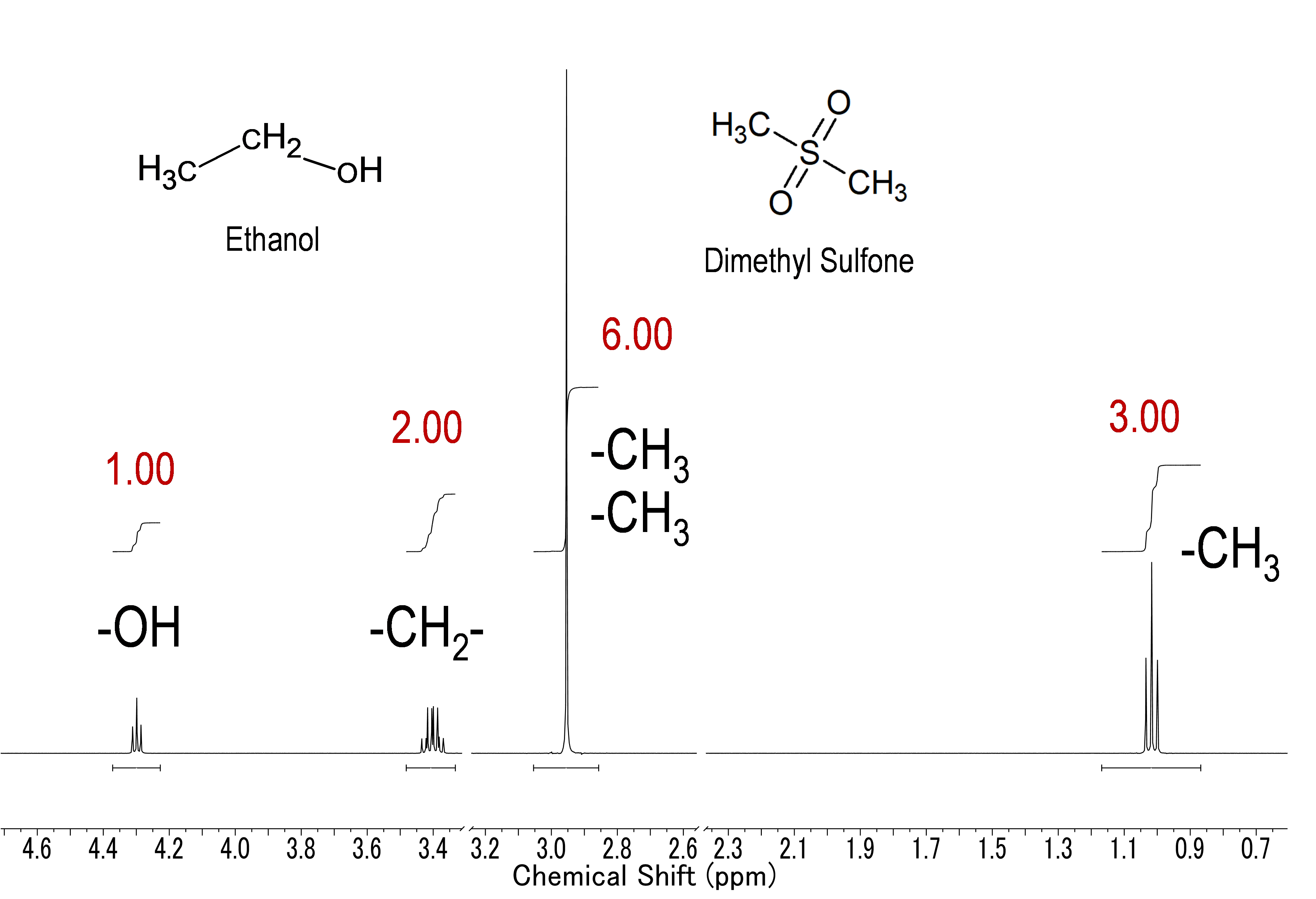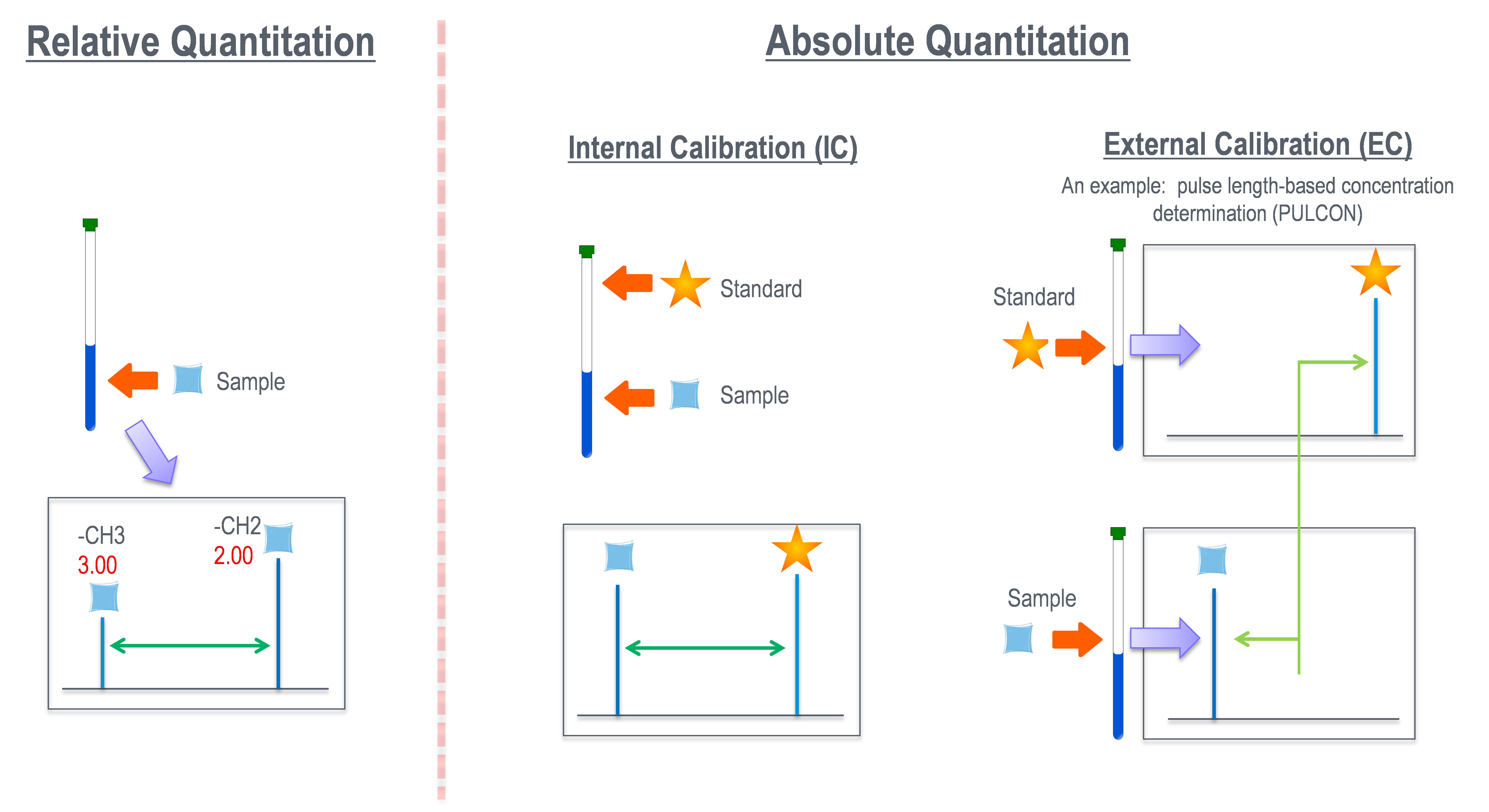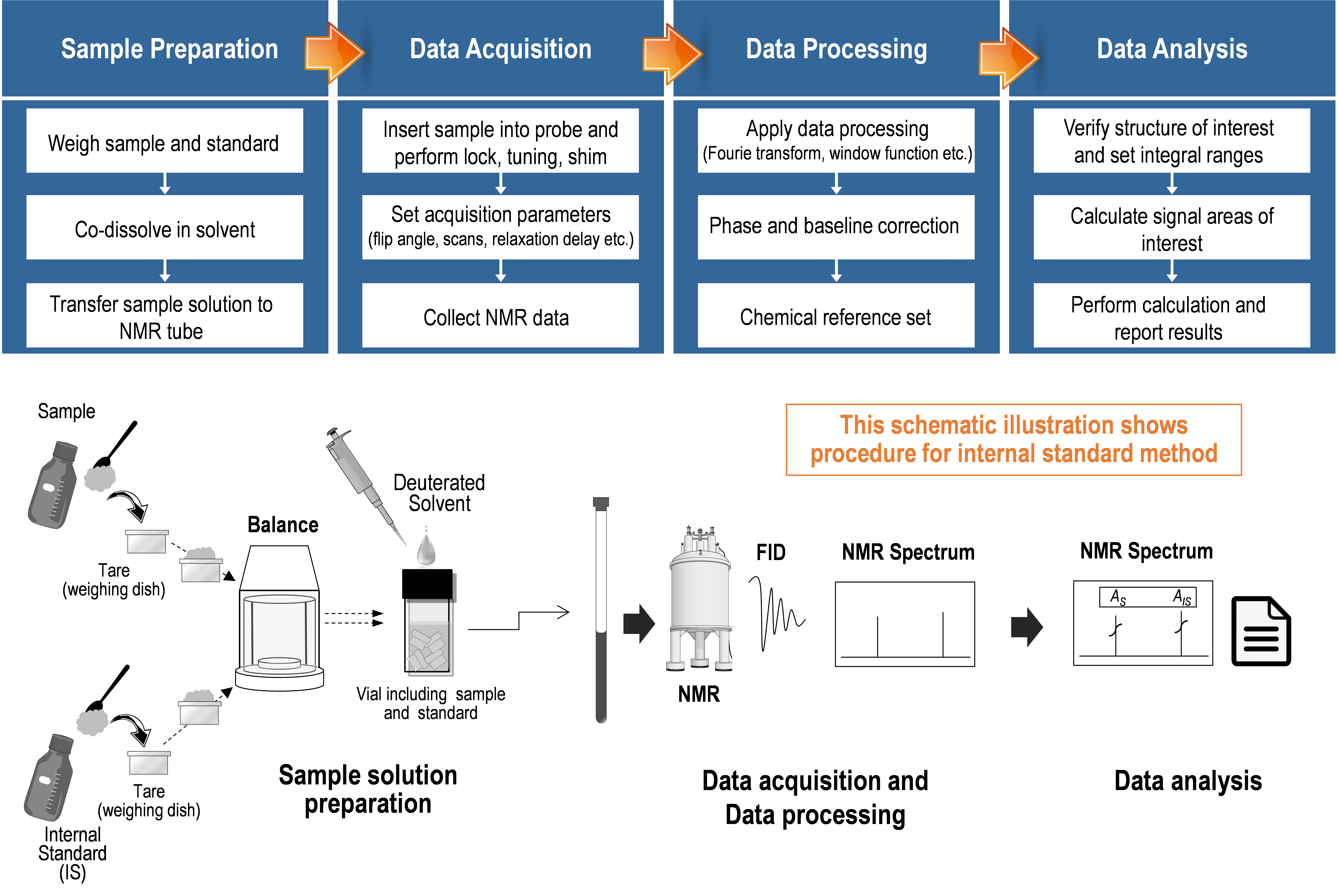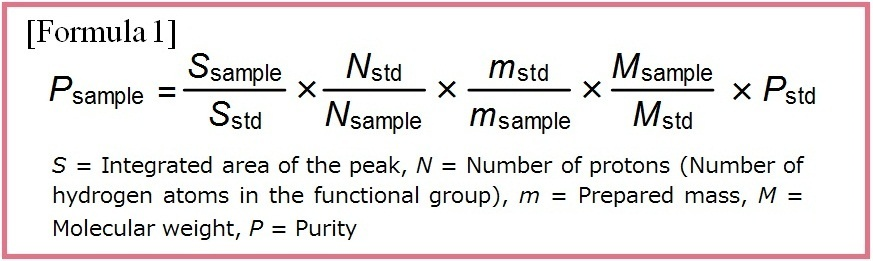# Quantitative NMR (qNMR)

Quantitative NMR (qNMR) is a quantitative technique using the physical utilizing the property that the ratio of the signal area observed on the NMR spectrum is proportional to the ratio of the number of nuclei in the molecule. This holds true whether the nuclei are part of the same molecular structure or located in different molecular entities. From the above, qNMR is possible to determine the mass fraction of an analyte with a substance different from the analyte as a standard for qNMR. In addition, quantification of a wide variety of compounds is theoretically possible using only one standard for qNMR as long as the signals of the analyte and the standard do not overlap each other.
In qNMR analysis, a mass fraction determination with metrological traceability is possible by using certified reference material (CRM) as a standard, calibrated balances for sample preparation, and evaluating measurement uncertainty.

## Product Line-up

### What is qNMR?In the mixture solution of ethanol and dimethyl sulfone in a molar ratio of 1: 1 (illustrated above), the ratio of the signal area is 3: 6: 2: 1. That is, the molar ratio of each component in the mixture solution can be determined by a simple ratio of normalized values, which are calculated by dividing the signal area by the number of nuclei. In the example above, the signal of methyl group of dimethyl sulfone has a signal area of 6 and the number of nuclei of 3, so 3 dividing by 3 gives a normalized signal area of 1. Similarly, the signal area of the methyl, methylene, and hydroxy groups of ethanol are 3, 2, and 1, and when normalized, they are all 1. Therefore, it can be seen that the molar ratio of ethanol to dimethyl sulfone is 1: 1. This simple example can be extended to much more complicated mixture solutions. Importantly, the internal standard (calibration standard) used in qNMR measurement does not have to be identical (the same type of substance) to the analyte. Therefore, with qNMR measurements, theoretically, it is possible to quantify all analytes using one type of internal standard (calibration standard). This important quantitative property makes qNMR a versatile technique that can be applied to many fields and compounds.

### Classification of qNMRAs mentioned above, NMR performs intra and intermolecular quantification, and the types can be classified into relative and absolute quantification. Relative quantification is used for verification of the chemical structure by confirming the number of nuclei of each functional group in the molecule, and confirmation of the mixture ratio. On the other hand, absolute quantification is used for measuring purity and concentration in the field of drug analysis and quality assurance. The above is the outline of the internal standard method in the absolute quantification, which is gradually beginning to be more widely used triggered by its application to the official analytical method, such as Japanese Pharmacopeia.

### General Workflow to Perform qNMR AnalysisqNMR procedures can be broadly divided into three parts (sample preparation, data acquisition (NMR measurement), and data processing) regardless of their type 1). Factors that cause bias or variability in the analytical results are potentially included in each part. However accurate analytical results can be obtained by using procedures suitable for qNMR.1. Miura T. et al. : Pham. Bull., 68(9), 868 (2020).

## Product Line-up

For research use or further manufacturing use only. Not for use in diagnostic procedures.

Product content may differ from the actual image due to minor specification changes etc.

If the revision of product standards and packaging standards has been made, there is a case where the actual product specifications and images are different.